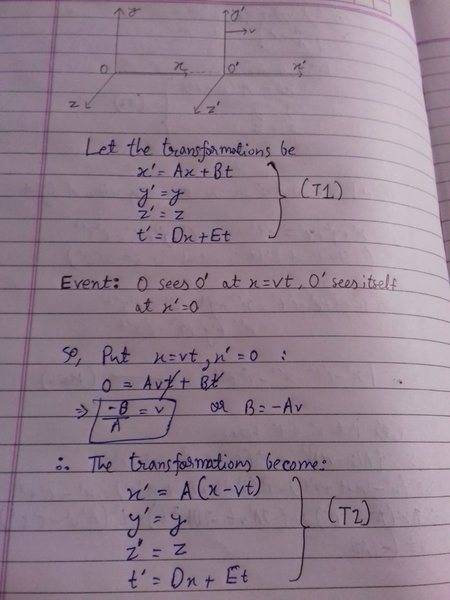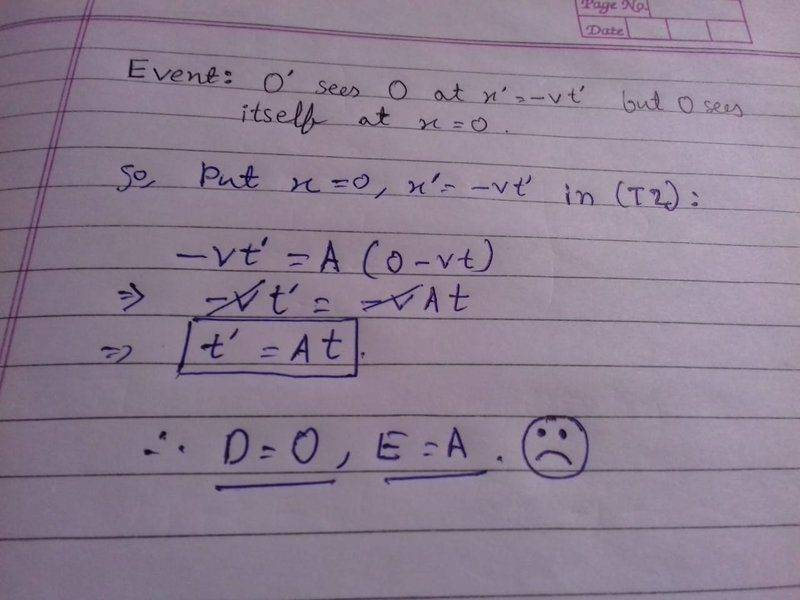# Doubt regarding derivation of Lorentz Transformations.

• I
• Kaguro
In summary, the conversation discusses the derivation of the Lorentz transformations in the Special Theory of Relativity. The assumptions made and the need for the invariance of the speed of light are explained. It is also noted that the assumptions in Newtonian mechanics lead to the Galilei transformation.

#### Kaguro

I have just started learning the Special Theory of Relativity. While deriving, I am facing some problems. I obviously have made some kind of mistake while using the equations...
What is wrong if I don't use the time transformation equation in Event #2?#### Attachments

Since y and z aren't involved, let's simplify to just talk about x and t.

Then a Lorentz transformation transforms a pair (x,t) into another pair, (x',t'). We're assuming that ##x' = Ax + Bt## and ##t' = Dx + Et##. Maybe it's clearer if we write them as functions:

##F_{x'}(x,t) = Ax + Bt##
##F_{t'}(x,t) = Dx + Et##

Then we have the assumptions:

Assumption 1: When ##x'=0##, ##x=vt##.

This means that the function ##F_{x'}(x,t)## satisfies ##F_{x'}(vt, t) = 0##. This implies that ##B = -vA##.

Assumption 2: When ##x=0##, ##x'=-vt'##.

This means that the functions ##F_{x'}(x,t)## and ##F_{t'}(x,t)## satisfy:

##F_{x'}(0, t) = -v F_{t'}(0, t)##.

So this implies that ##A\cdot 0 + B t = -v (D \cdot 0 + E t)##. So ##B = -vE##.

So you can conclude that ##E = A## and ##B = -vA##. But you can't conclude that ##D = 0##. To get ##D##, you need another assumption. That's usually the invariance of the speed of light:

Assumption 3: When ##x=ct##, then ##x' = c t'##.

In terms of functions,

##F_{x'}(ct, t) = c F_{t'}(ct, t)##

•Kaguro
stevendaryl said:
Since y and z aren't involved, let's simplify to just talk about x and t.

Then a Lorentz transformation transforms a pair (x,t) into another pair, (x',t'). We're assuming that ##x' = Ax + Bt## and ##t' = Dx + Et##. Maybe it's clearer if we write them as functions:

##F_{x'}(x,t) = Ax + Bt##
##F_{t'}(x,t) = Dx + Et##

Then we have the assumptions:

Assumption 1: When ##x'=0##, ##x=vt##.

This means that the function ##F_{x'}(x,t)## satisfies ##F_{x'}(vt, t) = 0##. This implies that ##B = -vA##.

Assumption 2: When ##x=0##, ##x'=-vt'##.

This means that the functions ##F_{x'}(x,t)## and ##F_{t'}(x,t)## satisfy:

##F_{x'}(0, t) = -v F_{t'}(0, t)##.

So this implies that ##A\cdot 0 + B t = -v (D \cdot 0 + E t)##. So ##B = -vE##.

So you can conclude that ##E = A## and ##B = -vA##. But you can't conclude that ##D = 0##. To get ##D##, you need another assumption. That's usually the invariance of the speed of light:

Assumption 3: When ##x=ct##, then ##x' = c t'##.

In terms of functions,

##F_{x'}(ct, t) = c F_{t'}(ct, t)##

Wow! Thank you very much! You explained very nicely. I was finally able to derive the Lorentz Transformations.

stevendaryl said:
So you can conclude that ##E = A## and ##B = -vA##. But you can't conclude that ##D = 0##. To get ##D##, you need another assumption. That's usually the invariance of the speed of light:

Assumption 3: When ##x=ct##, then ##x' = c t'##.

In terms of functions,

##F_{x'}(ct, t) = c F_{t'}(ct, t)##

Just to highlight the difference to Newtonian mechanics. A different assumption (and an incompatible one at that) would be ##t' = t##, which directly would imply that ##D = 0## and ##E = 1## and therefore leads to the Galilei transformation
$$x' = x - vt, \quad t' = t.$$
It is important to note that the special principle of relativity is still valid in Newtonian mechanics, what really changes when going to SR is the assumption that the speed of light is invariant replacing the assumption that there is a universal time.

•Kaguro